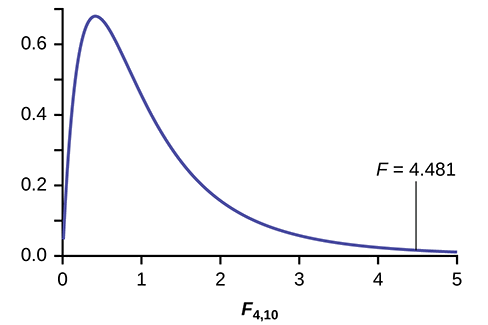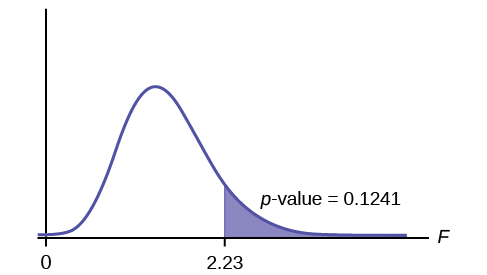# 13.4: Facts About the F Distribution

## Here are some facts about the $$F$$ distribution:

1. The curve is not symmetrical but skewed to the right.
2. There is a different curve for each set of $$dfs$$.
3. The $$F$$ statistic is greater than or equal to zero.
4. As the degrees of freedom for the numerator and for the denominator get larger, the curve approximates the normal.
5. Other uses for the $$F$$ distribution include comparing two variances and two-way Analysis of Variance. Two-Way Analysis is beyond the scope of this chapter.Figure $$\PageIndex{1}$$

Example $$\PageIndex{1}$$

Let’s return to the slicing tomato exercise. The means of the tomato yields under the five mulching conditions are represented by $$\mu_{1}, \mu_{2}, \mu_{3}, \mu_{4}, \mu_{5}$$. We will conduct a hypothesis test to determine if all means are the same or at least one is different. Using a significance level of 5%, test the null hypothesis that there is no difference in mean yields among the five groups against the alternative hypothesis that at least one mean is different from the rest.

The null and alternative hypotheses are:

• $$H_{0}: \mu_{1} = \mu_{2} = \mu_{3} = \mu_{4} = \mu_{5}$$
• $$H_{a}: \mu_{i} \neq \mu_{j}$$ some $$i \neq j$$

The one-way ANOVA results are shown in Table

one-way ANOVA results
Source of Variation Sum of Squares ($$SS$$) Degrees of Freedom ($$df$$) Mean Square ($$MS$$) $$F$$
Factor (Between) 36,648,561 $$5 - 1 = 4$$ $$\dfrac{36,648,561}{4} = 9,162,140$$

$$\dfrac{9,162,140}{2,044,672.6} = 4.4810$$

Error (Within) 20,446,726 $$15 - 5 = 10$$

$$\dfrac{20,446,726}{10} = 2,044,672.6$$

Total 57,095,287 $$15 - 1 = 14$$

Distribution for the test: $$F_{4,10}$$

$df(\text{num}) = 5 - 1 = 4$

$df(\text{denom}) = 15 - 5 = 10$

Test statistic: $$F = 4.4810$$Figure $$\PageIndex{2}$$

Probability Statement: $$p\text{-value} = P(F > 4.481) = 0.0248$$.

Compare $$\alpha$$ and the $$p\text{-value}$$: $$\alpha = 0.05, p\text{-value} = 0.0248$$

Make a decision: Since $$\alpha > p\text{-value}$$, we reject $$H_{0}$$.

Conclusion: At the 5% significance level, we have reasonably strong evidence that differences in mean yields for slicing tomato plants grown under different mulching conditions are unlikely to be due to chance alone. We may conclude that at least some of mulches led to different mean yields.

To find these results on the calculator:

Press STAT. Press 1:EDIT. Put the data into the lists L1, L2, L3, L4, L5.

Press STAT, and arrow over to TESTS, and arrow down to ANOVA. Press ENTER, and then enter L1, L2, L3, L4, L5). Press ENTER. You will see that the values in the foregoing ANOVA table are easily produced by the calculator, including the test statistic and the p-value of the test.

The calculator displays:

• $$F = 4.4810$$
• $$p = 0.0248$$ ($$p\text{-value}$$)

Factor

• $$df = 4$$
• $$SS = 36648560.9$$
• $$MS = 9162140.23$$

Error

• $$df = 10$$
• $$SS = 20446726$$
• $$MS = 2044672.6$$

Exercise $$\PageIndex{1}$$

MRSA, or Staphylococcus aureus, can cause a serious bacterial infections in hospital patients. Table shows various colony counts from different patients who may or may not have MRSA.

Conc = 0.6 Conc = 0.8 Conc = 1.0 Conc = 1.2 Conc = 1.4
9 16 22 30 27
66 93 147 199 168
98 82 120 148 132

Plot of the data for the different concentrations:Figure $$\PageIndex{3}$$

Test whether the mean number of colonies are the same or are different. Construct the ANOVA table (by hand or by using a TI-83, 83+, or 84+ calculator), find the p-value, and state your conclusion. Use a 5% significance level.

While there are differences in the spreads between the groups (Figure $$\PageIndex{1}$$), the differences do not appear to be big enough to cause concern.

We test for the equality of mean number of colonies:

$$H_{0}: \mu_{1} = \mu_{2} = \mu_{3} = \mu_{4} = \mu_{5}$$

$$H_{a}: \mu_{i} \neq \mu_{j}$$ some $$i \neq j$$

The one-way ANOVA table results are shown in Table.

Table $$\PageIndex{1}$$
Source of Variation Sum of Squares ($$SS$$) Degrees of Freedom ($$df$$) Mean Square ($$MS$$) $$F$$
Factor (Between) 10,233 $$5 - 1 = 4$$ $$\dfrac{10,233}{4} = 2,558.25$$ $$\dfrac{2,558.25}{4,194.9} = 0.6099$$
Error (Within) 41,949 $$15 - 5 = 10$$
Total 52,182 $$15 - 1 = 14$$ $$\dfrac{41,949}{10} = 4,194.9$$Figure $$\PageIndex{2}$$

Distribution for the test: $$F_{4,10}$$

Probability Statement: $$p\text{-value} = P(F > 0.6099) = 0.6649$$.

Compare $$\alpha$$ and the $$p\text{-value}$$: $$\alpha = 0.05, p\text{-value} = 0.669, \alpha > p\text{-value}$$

Make a decision: Since $$\alpha > p\text{-value}$$, we do not reject $$H_{0}$$.

Conclusion: At the 5% significance level, there is insufficient evidence from these data that different levels of tryptone will cause a significant difference in the mean number of bacterial colonies formed.

Example $$\PageIndex{2}$$

Four sororities took a random sample of sisters regarding their grade means for the past term. The results are shown in Table.

Figure $$\PageIndex{1}$$: MEAN GRADES FOR FOUR SORORITIES
Sorority 1 Sorority 2 Sorority 3 Sorority 4
2.17 2.63 2.63 3.79
1.85 1.77 3.78 3.45
2.83 3.25 4.00 3.08
1.69 1.86 2.55 2.26
3.33 2.21 2.45 3.18

Using a significance level of 1%, is there a difference in mean grades among the sororities?

Let $$\mu_{1}, \mu_{2}, \mu_{3}, \mu_{4}$$ be the population means of the sororities. Remember that the null hypothesis claims that the sorority groups are from the same normal distribution. The alternate hypothesis says that at least two of the sorority groups come from populations with different normal distributions. Notice that the four sample sizes are each five.

This is an example of a balanced design, because each factor (i.e., sorority) has the same number of observations.

$$H_{0}: \mu_{1} = \mu_{2} = \mu_{3} = \mu_{4}$$

$$H_{a}$$: Not all of the means $$\mu_{1}, \mu_{2}, \mu_{3}, \mu_{4}$$ are equal.

Distribution for the test: $$F_{3,16}$$

where $$k = 4$$ groups and $$n = 20$$ samples in total

$$df(\text{num}) = k - 1 = 4 - 1 = 3$$

$$df(\text{denom}) = n - k = 20 - 4 = 16$$

Calculate the test statistic: $$F = 2.23$$

Graph:Figure $$\PageIndex{5}$$

Probability statement: $$p\text{-value} = P(F > 2.23) = 0.1241$$

Compare $$\alpha$$ and the $$p\text{-value}$$: $$\alpha = 0.01$$

$$p\text{-value} = 0.1241$$

$$\alpha < p\text{-value}$$

Make a decision: Since $$\alpha < p\text{-value}$$, you cannot reject $$H_{0}$$.

Conclusion: There is not sufficient evidence to conclude that there is a difference among the mean grades for the sororities.

Put the data into lists L1, L2, L3, and L4. Press STAT and arrow over to TESTS. Arrow down to F:ANOVA. Press ENTER and Enter (L1,L2,L3,L4).

The calculator displays the F statistic, the $$p\text{-value}$$ and the values for the one-way ANOVA table:

$$F = 2.2303$$

$$p = 0.1241$$ ($$p\text{-value}$$)

Factor

$$df = 3$$

$$SS = 2.88732$$

$$MS = 0.96244$$

Error

$$df = 1$$

$$SS = 6.9044$$

$$MS = 0.431525$$

Exercise $$\PageIndex{2}$$

Four sports teams took a random sample of players regarding their GPAs for the last year. The results are shown in Table.

GPAs FOR FOUR SPORTS TEAMS

3.6 2.1 4.0 2.0
2.9 2.6 2.0 3.6
2.5 3.9 2.6 3.9
3.3 3.1 3.2 2.7
3.8 3.4 3.2 2.5

Use a significance level of 5%, and determine if there is a difference in GPA among the teams.

With a $$p\text{-value}$$ of $$0.9271$$, we decline to reject the null hypothesis. There is not sufficient evidence to conclude that there is a difference among the GPAs for the sports teams.

Example $$\PageIndex{3}$$

A fourth grade class is studying the environment. One of the assignments is to grow bean plants in different soils. Tommy chose to grow his bean plants in soil found outside his classroom mixed with dryer lint. Tara chose to grow her bean plants in potting soil bought at the local nursery. Nick chose to grow his bean plants in soil from his mother's garden. No chemicals were used on the plants, only water. They were grown inside the classroom next to a large window. Each child grew five plants. At the end of the growing period, each plant was measured, producing the data (in inches) in Table $$\PageIndex{3}$$.

Table $$\PageIndex{3}$$
Tommy's Plants Tara's Plants Nick's Plants
24 25 23
21 31 27
23 23 22
30 20 30
23 28 20

Does it appear that the three media in which the bean plants were grown produce the same mean height? Test at a 3% level of significance.

This time, we will perform the calculations that lead to the $$F'$$ statistic. Notice that each group has the same number of plants, so we will use the formula

$F' = \dfrac{n \cdot s_{\bar{x}}^{2}}{s^{2}_{\text{pooled}}}.$

First, calculate the sample mean and sample variance of each group.

Tommy's Plants Tara's Plants Nick's Plants
Sample Mean 24.2 25.4 24.4
Sample Variance 11.7 18.3 16.3

Next, calculate the variance of the three group means (Calculate the variance of 24.2, 25.4, and 24.4). Variance of the group means $$= 0.413 = s_{\bar{x}}^{2}$$

Then $$MS_{\text{between}} = ns_{\bar{x}}^{2} = (5)(0.413)$$ where $$n = 5$$ is the sample size (number of plants each child grew).

Calculate the mean of the three sample variances (Calculate the mean of 11.7, 18.3, and 16.3). Mean of the sample variances $$= 15.433 = s^{2}_{\text{pooled}}$$

Then $$MS_{\text{within}} = s^{2}_{\text{pooled}} = 15.433$$.

The $$F$$ statistic (or $$F$$ ratio) is $$F = \dfrac{MS_{\text{between}}}{MS_{\text{within}}} = \dfrac{ns_{\bar{x}}^{2}}{s^{2}_{\text{pooled}}} = \dfrac{(5)(0.413)}{15.433} = 0.134$$

The $$dfs$$ for the numerator $$= \text{the number of groups} - 1 = 3 - 1 = 2$$.

The $$dfs$$ for the denominator $$= \text{the total number of samples} - \text{the number of groups} = 15 - 3 = 12$$

The distribution for the test is $$F_{2,12}$$ and the $$F$$ statistic is $$F = 0.134$$

The $$p\text{-value}$$ is $$P(F > 0.134) = 0.8759$$.

Decision: Since $$\alpha = 0.03$$ and the $$p\text{-value} = 0.8759$$, do not reject $$H_{0}$$. (Why?)

Conclusion: With a 3% level of significance, from the sample data, the evidence is not sufficient to conclude that the mean heights of the bean plants are different.

To calculate the $$p\text{-value}$$:

*Press 2nd DISTR

*Arrow down to Fcdf(and press ENTER.

*Enter 0.134, E99, 2, 12)

*Press ENTER

The $$p\text{-value}$$ is $$0.8759$$.

Exercise $$\PageIndex{3}$$

Another fourth grader also grew bean plants, but this time in a jelly-like mass. The heights were (in inches) 24, 28, 25, 30, and 32. Do a one-way ANOVA test on the four groups. Are the heights of the bean plants different? Use the same method as shown in Example $$\PageIndex{3}$$.

• $$F = 0.9496$$
• $$p\text{-value} = 0.4402$$

From the sample data, the evidence is not sufficient to conclude that the mean heights of the bean plants are different.

Collaborative Exercise

From the class, create four groups of the same size as follows: men under 22, men at least 22, women under 22, women at least 22. Have each member of each group record the number of states in the United States he or she has visited. Run an ANOVA test to determine if the average number of states visited in the four groups are the same. Test at a 1% level of significance. Use one of the solution sheets in [link].

## References

1. Data from a fourth grade classroom in 1994 in a private K – 12 school in San Jose, CA.
2. Hand, D.J., F. Daly, A.D. Lunn, K.J. McConway, and E. Ostrowski. A Handbook of Small Datasets: Data for Fruitfly Fecundity. London: Chapman & Hall, 1994.
3. Hand, D.J., F. Daly, A.D. Lunn, K.J. McConway, and E. Ostrowski. A Handbook of Small Datasets.London: Chapman & Hall, 1994, pg. 50.
4. Hand, D.J., F. Daly, A.D. Lunn, K.J. McConway, and E. Ostrowski. A Handbook of Small Datasets. London: Chapman & Hall, 1994, pg. 118.
5. “MLB Standings – 2012.” Available online at http://espn.go.com/mlb/standings/_/year/2012.
6. Mackowiak, P. A., Wasserman, S. S., and Levine, M. M. (1992), "A Critical Appraisal of 98.6 Degrees F, the Upper Limit of the Normal Body Temperature, and Other Legacies of Carl Reinhold August Wunderlich," Journal of the American Medical Association, 268, 1578-1580.